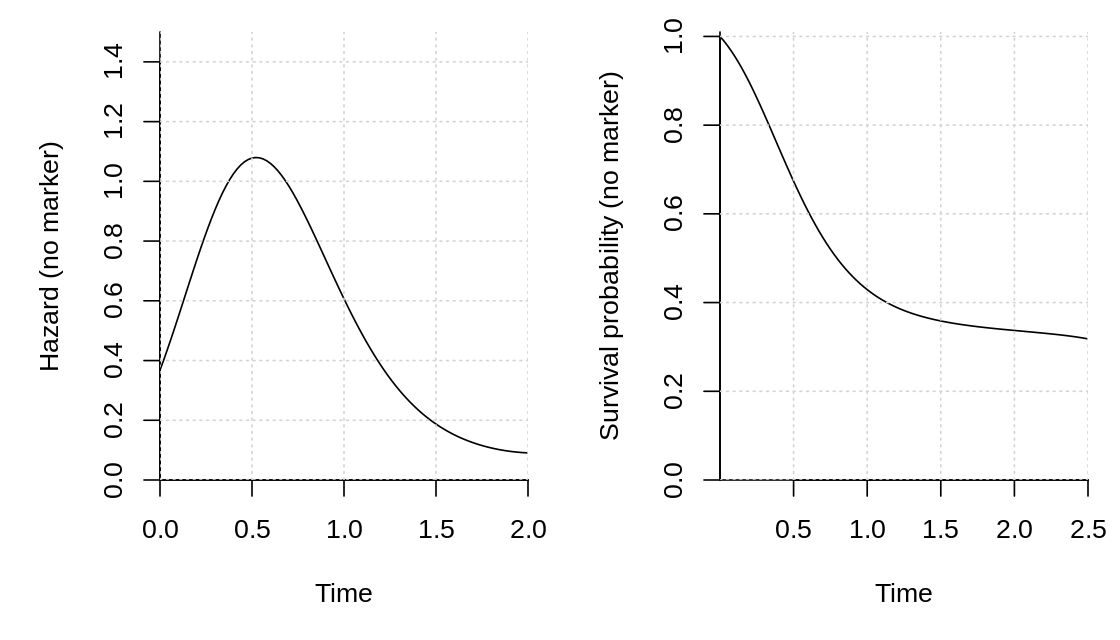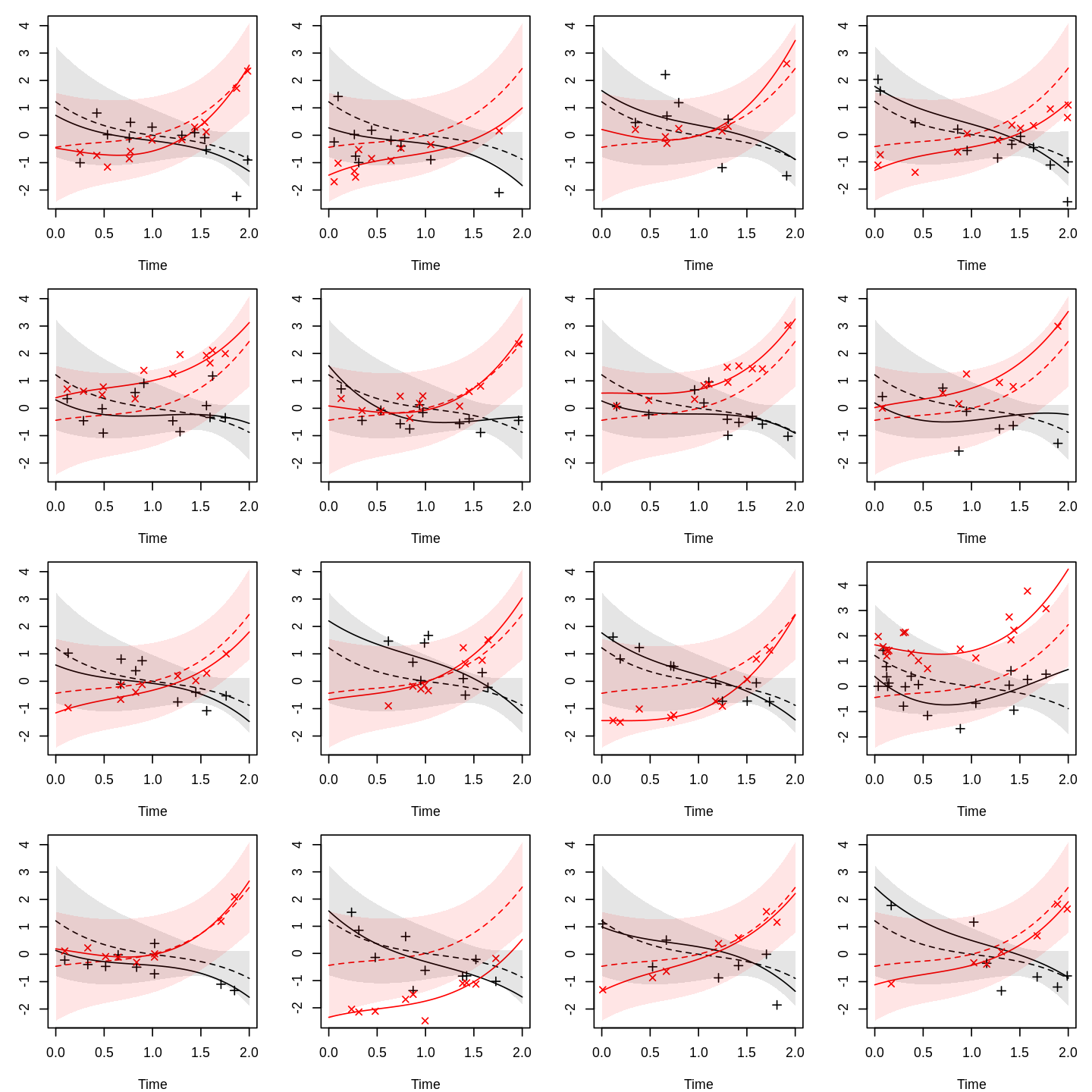# SimSurvNMarker: Simulate Survival time and Markers

The SimSurvNMarker package reasonably fast simulates from a joint survival and marker model. The package uses a combination of Gauss–Legendre quadrature and one dimensional root finding to simulate the event times as described by Crowther and Lambert (2013). Specifically, the package can simulate from the model

\begin{align*}\vec Y_{ij}\mid\vec U_i =\vec u_i &\sim N^{(r)}(\vec \mu_i(s_{ij}, \vec u_i), \Sigma)\\\vec\mu_i(s, \vec u)&=\Gamma^\top\vec x_i+B^\top\vec g(s)+U^\top\vec m(s)\\&=\left(I\otimes\vec x_i^\top\right)\text{vec}\Gamma+\left(I\otimes\vec g(s)^\top\right)\text{vec}B+\left(I\otimes\vec m(s)^\top\right)\vec u\\\vec U_i &\sim N^{(K)}(\vec 0,\Psi)\end{align*}

\begin{align*}h(t\mid\vec u)&=\exp\left(\vec\omega^\top\vec b(t)+\vec z_i^\top\vec\delta+\vec\alpha^\top\vec\mu_i(t, \vec u)\right)\\&=\exp\Bigg(\vec\omega^\top\vec b(t)+\vec z_i^\top\vec\delta+\vec 1^\top\left(\text{diag}(\vec\alpha)\otimes\vec x_i^\top\right)\text{vec}\Gamma+\vec 1^\top\left(\text{diag}(\vec\alpha)\otimes\vec g(t)^\top\right)\text{vec} B\\&\hspace{50pt}+\vec 1^\top\left(\text{diag}(\vec\alpha)\otimes\vec m(t)^\top\right)\vec u\Bigg)\end{align*}

where $$\vec Y_{ij}\in\mathbb R^{n_y}$$ is individual $$i$$’s $$j$$th observed marker at time $$s_{ij}$$, $$U_i\in\mathbb R^K$$ is individual $$i$$’s random effect, and $$h$$ is the instantaneous hazard rate for the time-to-event outcome. $$\vec\alpha$$ is the so-called association parameter. It shows the strength of the relation between the latent mean function, $$\vec\mu_i(t,\vec u)$$, and the log of the instantaneous rate, $$h(t\mid \vec u)$$. $$\vec m(t)$$, $$\vec g(t)$$ and $$\vec b(t)$$ are basis expansions of time. As an example, these can be a polynomial, a B-spline, or a natural cubic spline. The expansion for the baseline hazard, $$\vec b(t)$$, is typically made on $$\log t$$ instead of $$t$$. One reason is that the model reduces to a Weibull distribution when a first polynomial is used and $$\vec\alpha = \vec 0$$. $$\vec x_i$$ and $$\vec z_i$$ are individual specific known time-invariant covariates.

We provide an example of how to use the package here, in inst/test-data directory on Github, and at rpubs.com/boennecd/SimSurvNMarker-ex where we show that we simulate from the correct model by using a simulation study. The former examples includes

The purpose/goal of this package is to

• allow the user to specify all components in R including the basis functions.
• simulate reasonably fast from the model given this requirement.

## Installation

The package can be installed from Github using theremotes package:

stopifnot(require(remotes)) # needs the remotes package
install_github("boennecd/SimSurvNMarker")

It can also be installed from CRAN using install.packages:

install.packages("SimSurvNMarker")

## Example with Polynomials

We start with an example where we use polynomials as the basis functions. First, we assign the polynomial functions we will use.

b_func <- function(x){
x <- x - 1
cbind(x^3, x^2, x)
}
g_func <- function(x){
x <- x - 1
cbind(x^3, x^2, x)
}
m_func <- function(x){
x <- x - 1
cbind(x^2, x, 1)
}

We use a third order polynomial for the two fixed terms, $$\vec b$$ and $$\vec g$$, and a second order random polynomial for the random term, $$U_i^\top \vec m(s)$$, in the latent mean function. We choose the following parameters for the baseline hazard.

library(SimSurvNMarker)
omega <- c(1.4, -1.2, -2.1)
delta <- -.5 # the intercept

gl_dat <- get_gl_rule(30L)

# hazard function without marker
par(mar = c(5, 5, 1, 1), mfcol = c(1, 2))
plot(function(x) exp(drop(b_func(x) %*% omega) + delta),
xlim = c(0, 2), ylim = c(0, 1.5), xlab = "Time",
ylab = "Hazard (no marker)", xaxs = "i",  yaxs = "i", bty = "l")
grid()

# survival function without marker
plot(function(x) eval_surv_base_fun(x, omega = omega, b_func = b_func,
gl_dat = gl_dat, delta = delta),
xlim = c(1e-4, 2.5),
xlab = "Time", ylab = "Survival probability (no marker)", xaxs = "i",
yaxs = "i", bty = "l", ylim = c(0, 1.01))
grid()Then we set the following parameters for the random effect, $$\vec U_i$$, and the parameters for the marker process. We also simulate a number of latent markers’ mean curves and observed marker values and plot the result. The dashed curve is the mean, $$\vec\mu_i(s, \vec 0)$$, the fully drawn curve is the individual specific curve, $$\vec\mu_i(s, \vec U_i)$$, the shaded areas are a pointwise 95% interval for each mean curve, and the points are observed markers, $$\vec y_{ij}$$.

r_n_marker <- function(id)
# the number of markers is Poisson distributed
rpois(1, 10) + 1L
r_obs_time <- function(id, n_markes)
# the observations times are uniform distributed
sort(runif(n_markes, 0, 2))

Psi <- structure(c(0.18, 0.05, -0.05, 0.1, -0.02, 0.06, 0.05, 0.34, -0.25,
-0.06, -0.03, 0.29, -0.05, -0.25, 0.24, 0.04, 0.04,
-0.12, 0.1, -0.06, 0.04, 0.34, 0, -0.04, -0.02, -0.03,
0.04, 0, 0.1, -0.08, 0.06, 0.29, -0.12, -0.04, -0.08,
0.51), .Dim = c(6L, 6L))
B <- structure(c(-0.57, 0.17, -0.48, 0.58, 1, 0.86), .Dim = 3:2)
sig <- diag(c(.6, .3)^2)

# function to simulate a given number of individuals' markers' latent means
# and observed values
show_mark_mean <- function(B, Psi, sigma, m_func, g_func, ymax){
tis <- seq(0, ymax, length.out = 100)
Psi_chol <- chol(Psi)

on.exit(par(par_old))
par(mar = c(4, 3, 1, 1), mfcol = c(4, 4))

sigma_chol <- chol(sigma)
n_y <- NCOL(sigma_chol)
for(i in 1:16){
U <- draw_U(Psi_chol, n_y = n_y)
y_non_rng <- t(eval_marker(tis, B = B, g_func = g_func, U = NULL,
offset = NULL, m_func = m_func))
y_rng     <- t(eval_marker(tis, B = B, g_func = g_func, U = U,
offset = NULL, m_func = m_func))

sds <- sapply(tis, function(ti){
M <- (diag(n_y) %x% m_func(ti))
G <- (diag(n_y) %x% g_func(ti))
sds <- sqrt(diag(tcrossprod(M %*% Psi, M)))
cbind(drop(G %*% c(B)) - 1.96 * sds,
drop(G %*% c(B)) + 1.96 * sds)
}, simplify = "array")
lbs <- t(sds[, 1, ])
ubs <- t(sds[, 2, ])

y_obs <- sim_marker(B = B, U = U, sigma_chol = sigma_chol,
m_func = m_func, r_n_marker = r_n_marker,
r_obs_time = r_obs_time, g_func = g_func,
offset = NULL)

matplot(tis, y_non_rng, type = "l", lty = 2, ylab = "", xlab = "Time",
ylim = range(y_non_rng, y_rng, lbs, ubs, y_obs$y_obs)) matplot(tis, y_rng , type = "l", lty = 1, add = TRUE) matplot(y_obs$obs_time, y_obs\$y_obs, type = "p", add = TRUE, pch = 3:4)

polygon(c(tis, rev(tis)), c(lbs[, 1], rev(ubs[, 1])), border = NA,
col = rgb(0, 0, 0, .1))
polygon(c(tis, rev(tis)), c(lbs[, 2], rev(ubs[, 2])), border = NA,
col = rgb(1, 0, 0, .1))

}
invisible()
}
set.seed(1)
show_mark_mean(B = B, Psi = Psi, sigma = sig, m_func = m_func,
g_func = g_func, ymax = 2)As an example, we simulate the random effects and plot the conditional hazards and survival functions. We start by assigning the association parameter, $$\vec\alpha$$.

alpha <- c(.5, .9)

# function to plot simulated conditional hazards and survival
# functions
sim_surv_curves <- function(sig, Psi, delta, omega, alpha, B, m_func,
g_func, b_func, ymax) {
on.exit(par(par_old))
par(mfcol = c(1, 2), mar = c(5, 5, 1, 1))

# hazard functions
tis <- seq(0, ymax, length.out = 50)
n_y <- NCOL(sig)
Us <- replicate(100, draw_U(chol(Psi), n_y = n_y),
simplify = "array")

hz <- apply(Us, 3L, function(U)
vapply(tis, function(x)
exp(drop(delta + b_func(x) %*% omega +
alpha %*% eval_marker(ti = x, B = B, m_func = m_func,
g_func = g_func, U = U,
offset = NULL))),
FUN.VALUE = numeric(1L)))

matplot(tis, hz, lty = 1, type = "l",
col = rgb(0, 0, 0, .1), xaxs = "i", bty = "l", yaxs = "i",
ylim = c(0, max(hz, na.rm = TRUE)), xlab = "time",
ylab = "Hazard")
grid()

# survival functions
ys <- apply(Us, 3L, surv_func_joint,
ti = tis, B = B, omega = omega, delta = delta,
alpha = alpha, b_func = b_func, m_func = m_func,
gl_dat = gl_dat, g_func = g_func, offset = NULL)

matplot(tis, ys, lty = 1, type = "l", col = rgb(0, 0, 0, .1),
xaxs = "i", bty = "l", yaxs = "i", ylim = c(0, 1),
xlab = "time", ylab = "Survival probability")
grid()
}

set.seed(1)
sim_surv_curves(sig = sig, Psi = Psi, delta = delta, omega = omega,
alpha = alpha, B = B, m_func = m_func, g_func = g_func,
b_func = b_func, ymax = 2)